Next:10.4 The Classes P and NPUp:10.3 Examples of some Intractable ProblemsPrevious:10.3.5 Job Shop Scheduling

## 10.3.6 Satisfiability

A propositional variable is one that can be assigned the value true or false. A literal is a propositional variable or its negation. A clause is a sequence of literals separated by the logical OR operator. A propositional formula is said to be in conjunctive normal form (CNF) if it consists of a sequence of clauses separated by the logical AND operator. For example,

(p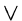qs)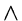(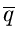r)(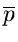r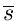)

A truth assignment for a set of propositional variables is an assignment of true or false value to each propositional variable. A truth assignment is said to satisfy a formula if it makes the value of the formula true.

3-SAT (Decision Problem): Given a CNF formula in which each clause is permitted to contain at most three literals, is there a truth assignment to its variables that satisfies it?

eEL,CSA_Dept,IISc,Bangalore